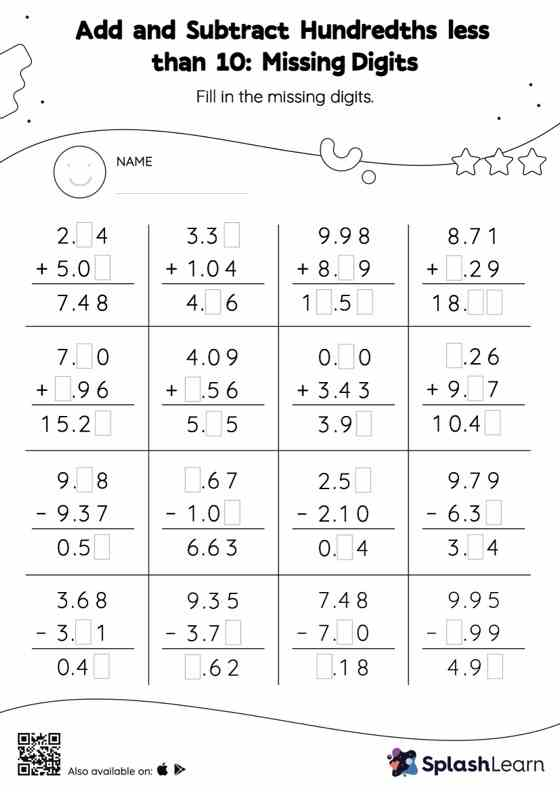# Add and Subtract Hundredths less than 10: Missing Digits Worksheet

Home > Add and Subtract Hundredths less than 10: Missing DigitsGive your little one some practice building addition and subtraction skills with this add and subtract hundredths less than 10 worksheet. In this worksheet, students add and subtract decimals by aligning the decimal points and using zero as a placeholder. They then use the relationship between addition and subtraction to find the missing number in the add and subtract hundredths less than 10 worksheet.